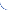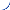定量给料机（皮带配料秤）简介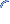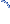DEL/DEM定量给料机系列

DEL/DEM调速定量给料秤（即皮带定量给料机或喂料机）是按德国SCHENCK技术生产的，集给料输送、称重计量和定量控制为一体，机电一体化的高技术产品。能适应各种工业生产环境的粉、粒、块状散体物料连续给料计量和自动控制配料系统。广泛地应用于建材、冶金、电力、化工、轻工等工业生产部门

主要性能参数如下：

温度：仪表-50～50℃；

秤体-30～50℃；

相对湿度≤90％。

电源：～220V/50Hz（仪表部分）。

DEL／DEM型定量给料机在机械结构上还有下面一些特点。

1、皮带更换容易。只要张紧装置松开皮带，移动一侧支架,即可取下皮带予以更换。

2、计量精度受皮带影响小。由于有皮带自动张紧装置，张力基本恒定，兼之称重传感器最大位移量仅 0.2mm，故皮带张力对计量精度影响可以忽略不计。

3、对于不同物料或不同工艺要求，可采用不同的给料料斗，并有挡料板和配套的卸料溜子。

4、皮带和滚筒之间有犁形清扫器，皮带外面有刮板，并带有橡胶刀口，可清除皮带面上粘附的物料。

5、在皮带两侧有皮带限位开关，当皮带跑偏触动限位开关时，通过微机发出报警信号。

6、在机架上有皮带张紧标准刻度，能很方便地进行皮带张紧调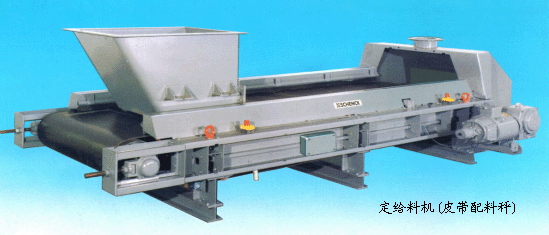1、适用于单机和DCS集散型控制系统。结构简单、价格便宜。大中小型企业均可采用。

2、先进的微处理机技术，线性化数字处理和 PID调节方式，确保运行可靠；响应快，控制稳定。

3、信号现场处理，以电流方式输出和抗干扰技术，确保抗干扰性强，系统长期稳定。

4、自动检测和自动调零去皮重。确保较高给料计量精度。

5、多种标准信号通讯，多种控制方式，可方便的与DCS控制系统相连。

6、具有手动／自动操作、掉电保护、越限报警等全面功能。操作、调试、维护简单方便。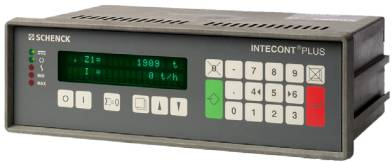集中控制管理系统的主要特点：

1、适用于3－8台或更多台给料秤的给料计量和配料系统的集中控制。可靠性高、功能强、操作直观方便。

2、以工控机为硬环境，配以本研究所的模块化软件系统，稳定可靠，功能全面。

3、融管理和过程控制为一体，全汉字界面，多画面显示运行状态一目了然；操作、修改参数简单方便。

4、设有标准输入／输出接口，可以模拟信号或标准串口等协议方式通讯。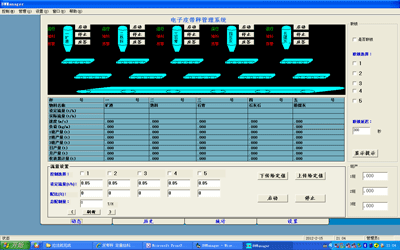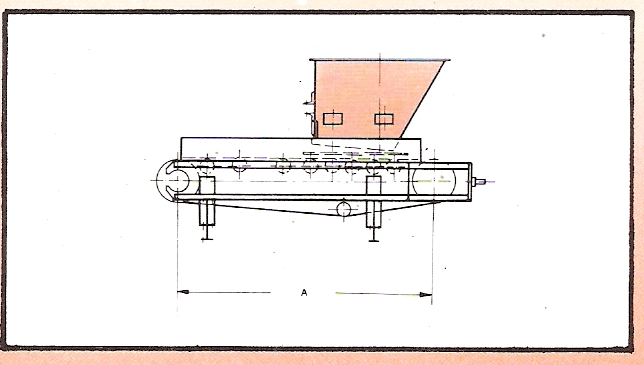用于自由流动物料的给料料斗(T型,T4,T6,T20),料斗与料仓出口用法兰连接,料斗有垂直出口延伸到挡板底部,手动或旋转式开关闸门 型号 滚筒中心距A(mm) DEL 1300 2000 2700 3500 4000 4500 5000 DEM 1500 2000 2700 3500 4000 4500 5000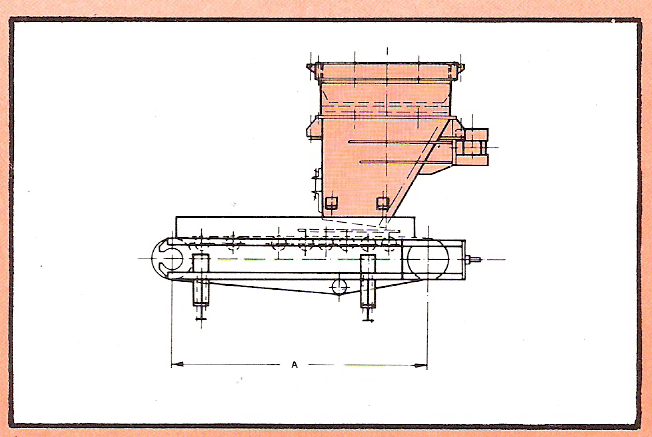用于流动性差的物料的振动给料料斗(V型,V2,V5),悬架的入口套管与料连接,给料斗上装有振动器,垂直出口及闸门,振动器与料仓及给料器之间是隔振的 型号 滚筒中心距A(mm) DEL 1300 2000 2700 3500 4000 4500 5000 DEM 1500 2000 2700 3500 4000 4500 5000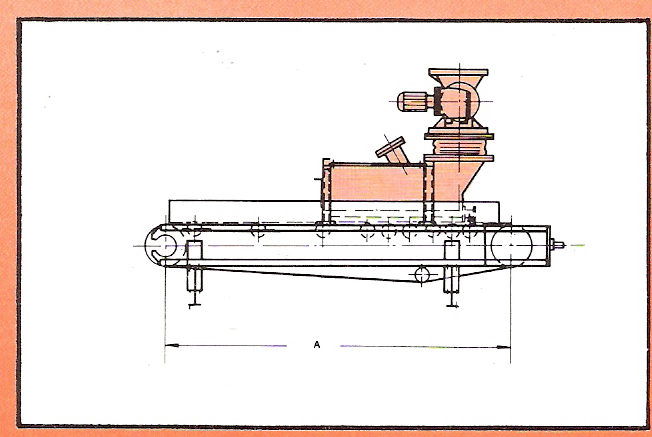用于倾泻性物料流的星型给料器及S型料斗,星型给料器由三相交流电机驱动,为给料料斗进行间歇式填充. 型号 滚筒中心距A(mm) DEL -- -- 2700 3500 4000 4500 5000 DEM -- -- 2700 3500 4000 4500 5000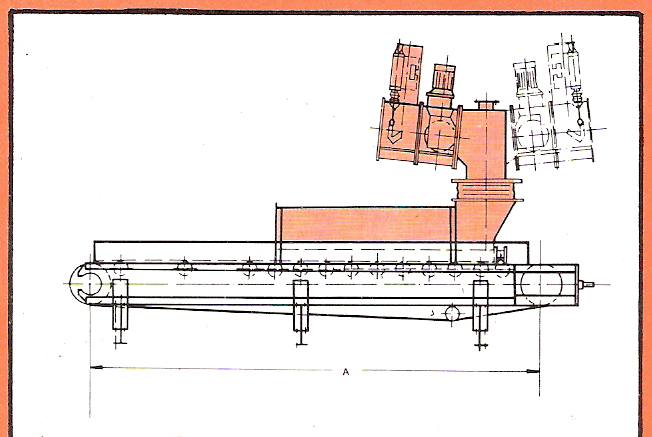用于流体化散料的气动截止阀、电动调节阀及S型料斗，进料口的开关由齿轮式位置控制电机来控制使给料器控制在设定值附近。断电或称重给料器停机时，通往气源的闸门将自动关闭。 型号 滚筒中心距A(mm) DEL -- -- -- 3500 4000 4500 5000 DEM -- -- -- 3500 4000 4500 5000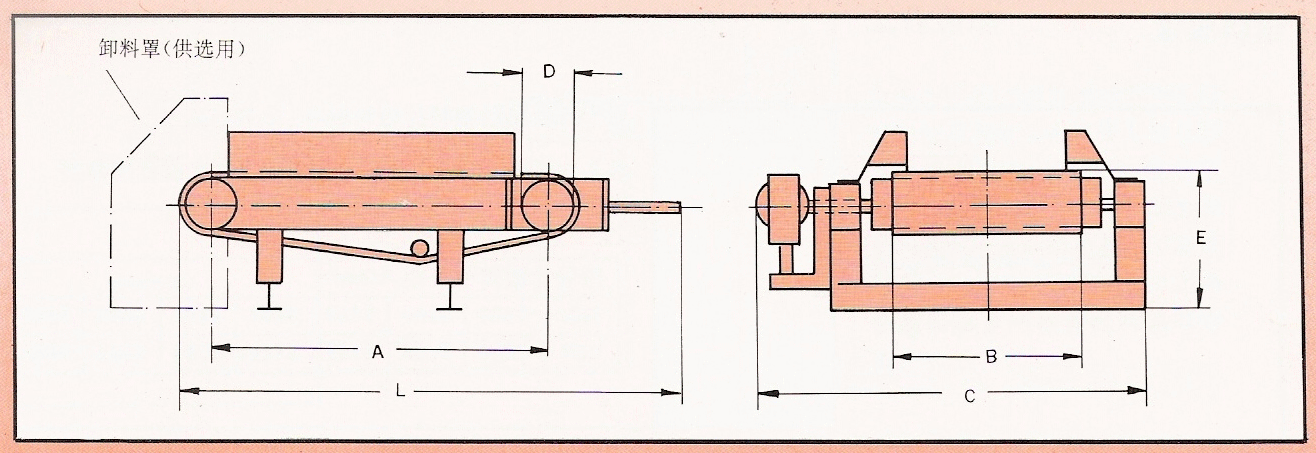型号 滚筒中心距A(mm) 皮带宽度B（mm) 650 800 1000 1400 1600 1800 DEL 1300 L=1850 G=1320 L=1820 C=1520 2000 L=2520 C=1320 L=2520 C=1520 L=2520 C=1720 2700 L=3220 C=1320 L=3220 C=1520 L=2520 C=1720 3500 L=4020 C=1320 L=4020 C=1520 L=4020 C=1720 4000 L=4520 C=1320 L=4520 C=1520 L=4520 C=1720 4500 L=2520 C=1320 L=2520 C=1520 L=2520 C=1720 5000 L=5520 C=1320 L=5520 C=1520 L=5520 C=1720 DEM 1500 L=21900 C=1860 L=2190 C=2110 2000 L=26900 C=1860 L=2190 C=2110 L=2690 C=2310 2700 L=3390 C=1860 L=3390 C=2110 L=3390 C=2310 3500 L=4190 C=1860 L=4190 C=2110 L=4190 C=2310 L=4190 C=2510 4000 L=4690 C=1860 L=4690 C=2110 L=4690 C=2310 L=4690 C=5510 4500 L=5190 C=1860 L=5190 C=2110 L=5190 C=2310 L=5190 C=2510 5000 L=5690 C=1860 L=5690 C=2110 L=5690 C=2310 L=5690 C=2510